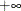# The EXPAND Procedure

### Transforming Series

The interpolation methods used by PROC EXPAND assume that there are no restrictions on the range of values that series can have. This assumption can sometimes cause problems if the series must be within a certain range.

For example, suppose you are converting monthly sales figures to weekly estimates. Sales estimates should never be less than zero, but since the spline curve ignores this restriction some interpolated values may be negative. One way to deal with this problem is to transform the input series before fitting the interpolating spline and then reverse transform the output series.

You can apply various transformations to the input series using the TRANSFORMIN= option on the CONVERT statement. (The TRANSFORMIN= option can be abbreviated as TRANSFORM= or TIN=.) You can apply transformations to the output series using the TRANSFORMOUT= option. (The TRANSFORMOUT= option can be abbreviated as TOUT=.)

For example, you might use a logarithmic transformation of the input sales series and exponentiate the interpolated output series. The following statements fit a spline curve to the log of SALES and then exponentiate the output series.

   proc expand data=a out=b from=month to=week;
id date;
convert sales / observed=total
transformin=(log)
transformout=(exp);
run;


Note that the transformations specified by the TRANSFORMIN= option are applied before the data are interpolated; the cubic spline curve or other interpolation method is fitted to transformed input data. The transformations specified by the TRANSFORMOUT= option are applied to interpolated values computed from the curves fit to the transformed input data.

As another example, suppose you are interpolating missing values in a series of market share estimates. Market shares must be between 0% and 100%, but applying a spline interpolation to the raw series can produce estimates outside of this range.

The following statements use the logistic transformation to transform proportions in the range 0 to 1 to values in the rangeto. The TIN= option first divides the market shares by 100 to rescale percent values to proportions and then applies the LOGIT function. The TOUT= option applies the inverse logistic function ILOGIT to the interpolated values to convert back to proportions and then multiplies by 100 to rescale back to percentages.

   proc expand data=a out=b;
id date;
convert mshare / tin=( / 100 logit )
tout=( ilogit * 100 );
run;


When more than one transformation is specified in the TRANSFORMIN= or TRANSFORMOUT= option, the transformations are applied in the order in which they are listed. Thus in the above example the complete input transformation is logit(mshare/100) (and not logit(mshare)/100) because the division operation is listed first in the TIN= option.

You can also use the TRANSFORM= (or TRANSFORMOUT=) option as a convenient way to do calculations normally performed with the SAS DATA step. For example, the following statements add the lead of X to the data set A. The METHOD=NONE option is used to suppress interpolation.

   proc expand data=a method=none;
id date;
convert x=xlead / transform=(lead);
run;


Any number of operations can be listed in the TRANSFORMIN= and TRANSFORMOUT= options. See Table 15.1 for a list of the operations supported.